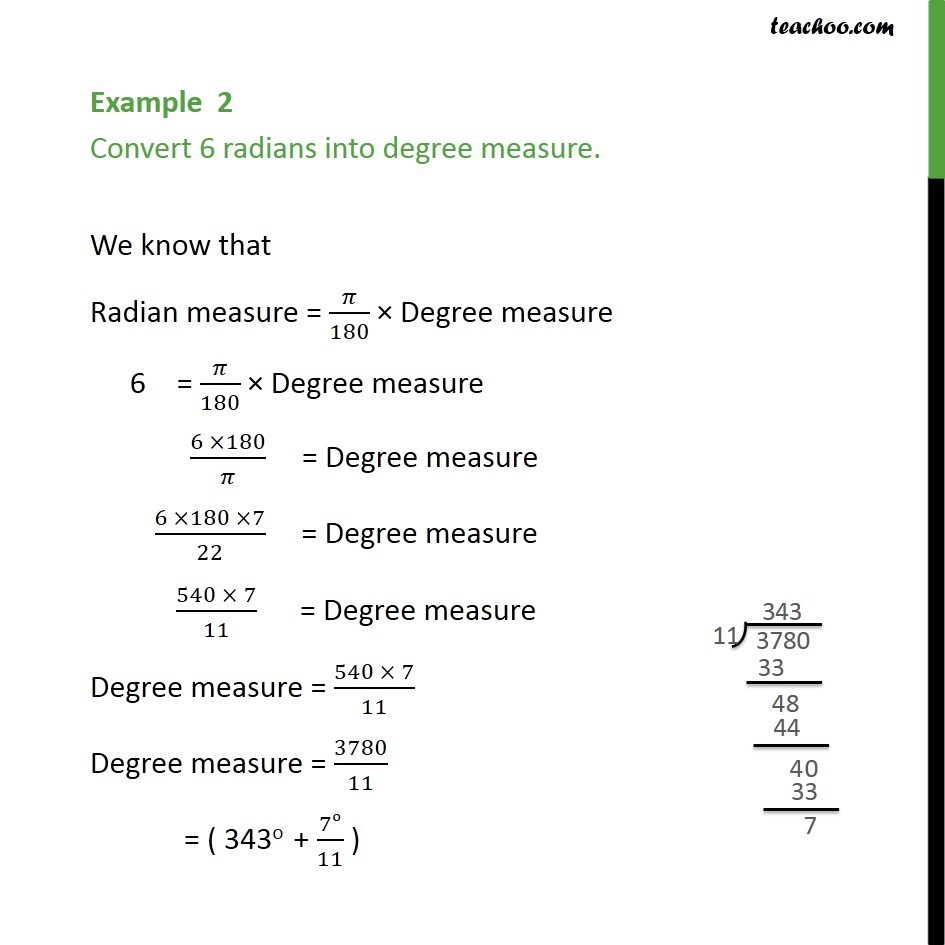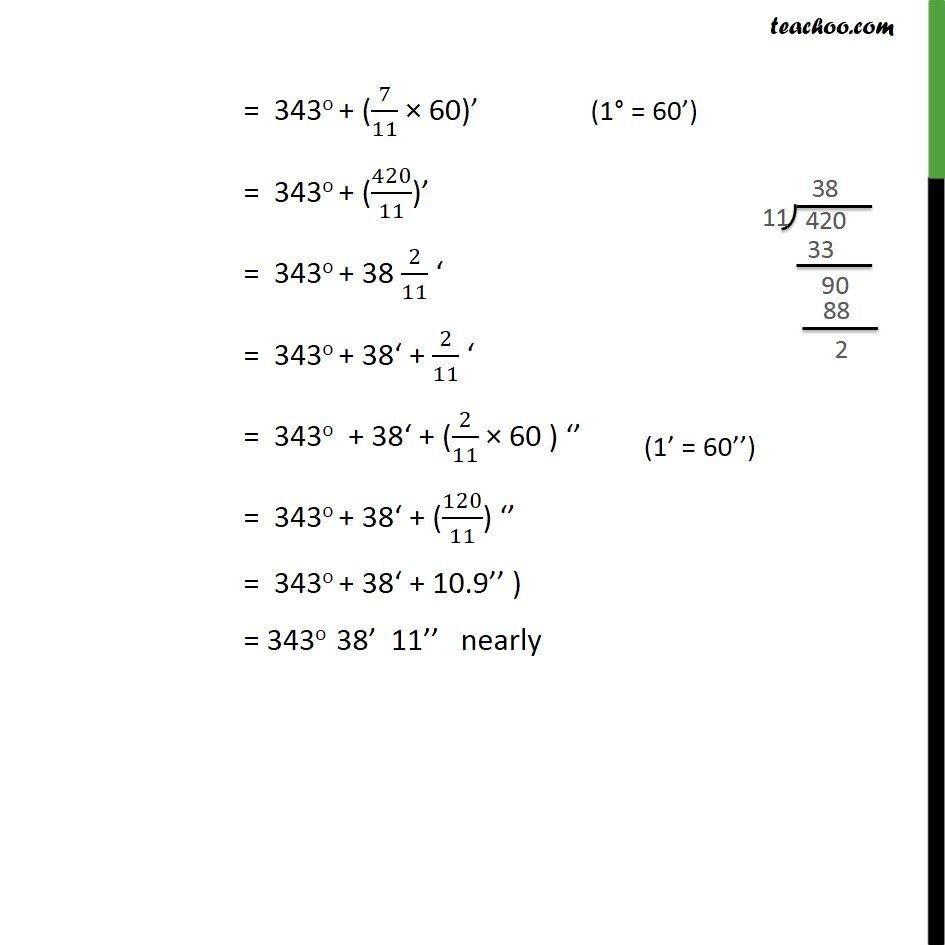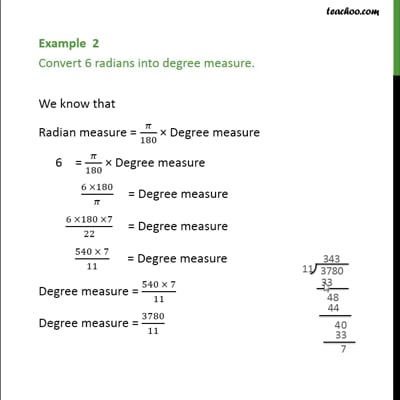Chapter 3 Class 11 Trigonometric Functions
Concept wiseThis video is only available for Teachoo black users

Introducing your new favourite teacher - Teachoo Black, at only ₹83 per month

### Transcript

Example 2 Convert 6 radians into degree measure. We know that Radian measure = /180 Degree measure 6 = /180 Degree measure (6 180)/ = Degree measure (6 180 7)/22 = Degree measure (540 7)/11 = Degree measure Degree measure = (540 7)/11 Degree measure = 3780/11 = ( 343o + 7o/11 ) = 343o + (7/11 60) = 343o + (420/11) = 343o + 38 2/11 = 343o + 38 + 2/11 = 343o + 38 + (2/11 60 ) = 343o + 38 + (120/11) = 343o + 38 + 10.9 ) = 343o 38 11 nearly